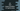# C# program to find the largest and smallest numbers in an user given array## C# program to find the largest and smallest numbers in an user given array:

In this post, we will learn how to find the largest and smallest numbers in an user given array. Our program will ask the user to enter the size of the array. It will then take the numbers as inputs from the user one by one. Finally, we will iterate through the array one more time to find the largest and smallest.

### C# program:

Below is the complete C# program:

``````using System;

public class Program
{
public static void Main()
{
//1
Console.WriteLine("Enter the size of the array :");

//2
int[] arr = new int[size];

//3
for(int i =0 ; i<size; i++){
Console.WriteLine("Enter value for index "+i+" : ");
}

//4
int largest = arr;
int smallest = arr;

//5
for(int i=1; i<size; i++){
if(arr[i] > largest){
largest = arr[i];
}
if(arr[i] < smallest){
smallest = arr[i];
}
}

//6
Console.WriteLine("Smallest value : "+smallest+", and largest value : "+largest);
}
}``````

### Explanation:

The commented numbers in the above program denote the step numbers below :

1. Ask the user to enter the size of the array. Read it and store it in the integer variable size.
2. Create one new integer array arr to store the user input values. It is of size size.
3. Run one for loop to read the values for the array. Ask the user to enter the value for each index of the array. Read it and store it in that index of the array.
4. Create two int variables to hold the largest and smallest values in the array. Assign the first element of the array to both of these variables. We will compare all other elements of the array with the current value of these variables to find out the largest and smallest.
5. We are running one more for loop to find the smallest and largest numbers in the array. We are comparing the current value of smallest and largest with all elements from index 1 to the end. If we find any element that is smaller than smallest, we assign it as smallest. Again, if we find any element that is greater than greatest, we assign it as largest.
6. Finally, we are printing the smallest and largest values of the array.

### Sample output:

``````Enter the size of the array :
5
Enter value for index 0 :
1
Enter value for index 1 :
10
Enter value for index 2 :
20
Enter value for index 3 :
30
Enter value for index 4 :
13
Smallest value : 1, and largest value : 30``````